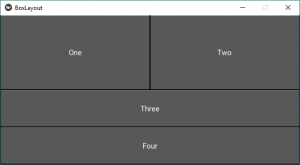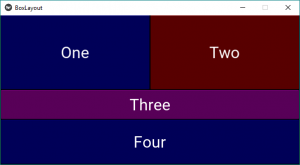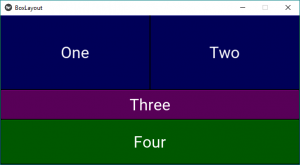GFG App
Open AppBrowser
Continue

# Python | BoxLayout widget in Kivy

Kivy is a platform independent GUI tool in Python. As it can be run on Android, IOS, Linux and Windows, etc. It is basically used to develop the Android application, but it does not mean that it can not be used on Desktops applications.

Now in this article, we will learn about the use of BoxLayout widget in kivy and how to add some features like color, size etc to it.
BoxLayout:
BoxLayout arranges widgets in either in a vertical fashion that is one on top of another or in a horizontal fashion that is one after another. When you will not provide any size-hint then the child widgets divides the size of its parent widget equally or accordingly.

Basic Approach to follow while creating button :
1) import kivy
2) import kivyApp
3) import BoxLayout
4) set minimum version(optional)
6) Extend the class
7) Return layout
8) Run an instance of the class

Below is the code to use BoxLayout:

## Python3

 `# code to show how to use nested boxlayouts.`   `# import kivy module ` `import` `kivy ` `  `  `# this restricts the kivy version i.e ` `# below this kivy version you cannot ` `# use the app or software ` `kivy.require(``"1.9.1"``) ` `  `  `# base Class of your App inherits from the App class. ` `# app:always refers to the instance of your application ` `from` `kivy.app ``import` `App ` `  `  `# creates the button in kivy ` `# if not imported shows the error ` `from` `kivy.uix.button ``import` `Button`   `# BoxLayout arranges children in a vertical or horizontal box.` `# or help to put the children at the desired location.` `from` `kivy.uix.boxlayout ``import` `BoxLayout` `  `  `# class in which we are creating the button by using boxlayout ` `# defining the App class` `class` `BoxLayoutApp(App): ` `      `  `    ``def` `build(``self``):`   `        ``# To position oriented widgets again in the proper orientation ` `        ``# use of vertical orientation to set all widgets  ` `        ``superBox ``=` `BoxLayout(orientation ``=``'vertical'``)`   `        ``# To position widgets next to each other,` `        ``# use a horizontal BoxLayout.` `        ``HB ``=` `BoxLayout(orientation ``=``'horizontal'``)` `        `  `        ``btn1 ``=` `Button(text ``=``"One"``)` `        ``btn2 ``=` `Button(text ``=``"Two"``)`   `        ``# HB represents the horizontal boxlayout orientation` `        ``# declared above` `        ``HB.add_widget(btn1)` `        ``HB.add_widget(btn2)`   `        ``# To position widgets above/below each other,` `        ``# use a vertical BoxLayout.` `        ``VB ``=` `BoxLayout(orientation ``=``'vertical'``)`   `        ``btn3 ``=` `Button(text ``=``"Three"``)` `        ``btn4 ``=` `Button(text ``=``"Four"``)`   `        ``# VB represents the vertical boxlayout orientation` `        ``# declared above` `        ``VB.add_widget(btn3)` `        ``VB.add_widget(btn4)`   `        ``# superbox used to again align the oriented widgets` `        ``superBox.add_widget(HB)` `        ``superBox.add_widget(VB)`   `        ``return` `superBox`   `# creating the object root for BoxLayoutApp() class  ` `root ``=` `BoxLayoutApp() ` `  `  `# run function runs the whole program ` `# i.e run() method which calls the ` `# target function passed to the constructor. ` `root.run() `

Output:Some modification like add colour, fix size in Box layout widget:

## Python3

 `# code to show how to use nested boxlayouts.`   `# import kivy module ` `import` `kivy `   `# to choose the colors randomly` `# every time you run it shows different color` `import` `random  `   `# this restricts the kivy version i.e ` `# below this kivy version you cannot ` `# use the app or software ` `kivy.require(``"1.9.1"``) ` `  `  `# base Class of your App inherits from the App class. ` `# app:always refers to the instance of your application ` `from` `kivy.app ``import` `App ` `  `  `# creates the button in kivy ` `# if not imported shows the error ` `from` `kivy.uix.button ``import` `Button`   `# BoxLayout arranges children in a vertical or horizontal box.` `# or help to put the children at the desired location.` `from` `kivy.uix.boxlayout ``import` `BoxLayout`   `# declaring the colours you can use directly also` `red ``=` `[``1``, ``0``, ``0``, ``1``]` `green ``=` `[``0``, ``1``, ``0``, ``1``]` `blue ``=`  `[``0``, ``0``, ``1``, ``1``]` `purple ``=` `[``1``, ``0``, ``1``, ``1``]` `  `  `# class in which we are creating the button ` `class` `BoxLayoutApp(App): ` `      `  `    ``def` `build(``self``):`   `        ``# To position oriented widgets again in the proper orientation ` `        ``# use of vertical orientation to set all widgets  ` `        ``superBox ``=` `BoxLayout(orientation ``=``'vertical'``)`   `        ``# To position widgets next to each other,` `        ``# use a horizontal BoxLayout.` `        ``HB ``=` `BoxLayout(orientation ``=``'horizontal'``)`   `        ``colors ``=` `[red, green, blue, purple]` `        `  `        ``# styling the button boxlayout` `        ``btn1 ``=` `Button(text ``=``"One"``,` `                      ``background_color ``=` `random.choice(colors),` `                      ``font_size ``=` `32``,` `                      ``size_hint ``=``(``0.7``, ``1``))` `        ``btn2 ``=` `Button(text ``=``"Two"``,` `                      ``background_color ``=` `random.choice(colors),` `                      ``font_size ``=` `32``,` `                      ``size_hint ``=``(``0.7``, ``1``))`   `        ``# HB represents the horizontal boxlayout orientation` `        ``# declared above` `        ``HB.add_widget(btn1)` `        ``HB.add_widget(btn2)`   `        ``# To position widgets above/below each other,` `        ``# use a vertical BoxLayout.` `        ``VB ``=` `BoxLayout(orientation ``=``'vertical'``)`   `        ``btn3 ``=` `Button(text ``=``"Three"``,` `                      ``background_color ``=` `random.choice(colors),` `                      ``font_size ``=` `32``,` `                      ``size_hint ``=``(``1``, ``10``))` `        ``btn4 ``=` `Button(text ``=``"Four"``,` `                      ``background_color ``=` `random.choice(colors),` `                      ``font_size ``=` `32``,` `                      ``size_hint ``=``(``1``, ``15``))`   `        ``# VB represents the vertical boxlayout orientation` `        ``# declared above` `        ``VB.add_widget(btn3)` `        ``VB.add_widget(btn4)`   `        ``# superbox used to again align the oriented widgets` `        ``superBox.add_widget(HB)` `        ``superBox.add_widget(VB)`   `        ``return` `superBox`   `# creating the object root for BoxLayoutApp() class  ` `root ``=` `BoxLayoutApp() ` `  `  `# run function runs the whole program ` `# i.e run() method which calls the ` `# target function passed to the constructor. ` `root.run() `

Output:
Picture1:Picture2:Note: Every time you run the code it provides you the different color outputs as in the pictures.

My Personal Notes arrow_drop_up